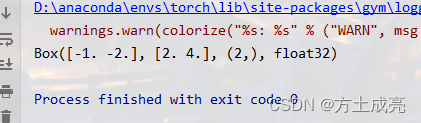﻿ Python的Gym库中的Box类

# 一文李青 理清Gym中的Box类

Box的部分官方代码如下：

``````import numpy as np
import warnings

from .space import Space
from gym import logger

class Box(Space):
"""
A (possibly unbounded) box in R^n. Specifically, a Box represents the
Cartesian product of n closed intervals. Each interval has the form of one
of [a, b], (-oo, b], [a, oo), or (-oo, oo).

There are two common use cases:

* Identical bound for each dimension::
>>> Box(low=-1.0, high=2.0, shape=(3, 4), dtype=np.float32)
Box(3, 4)

* Independent bound for each dimension::
>>> Box(low=np.array([-1.0, -2.0]), high=np.array([2.0, 4.0]), dtype=np.float32)
Box(2,)

"""
``````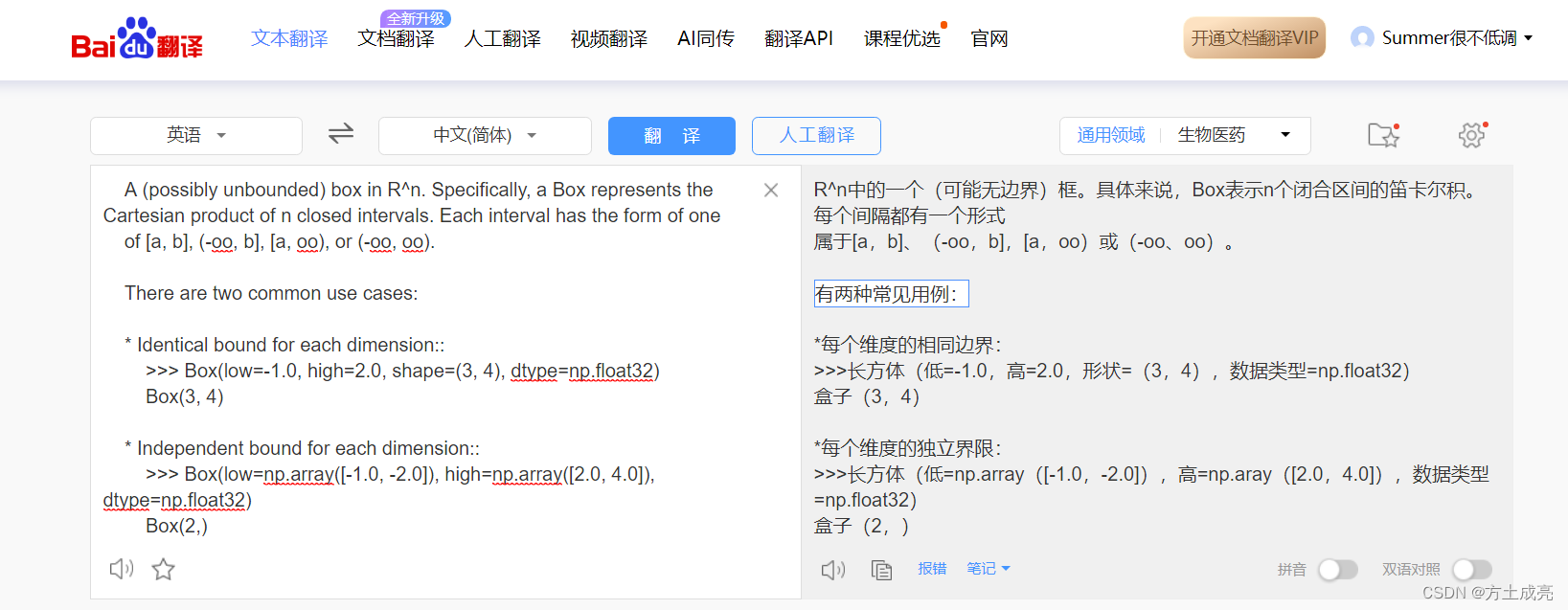box数据类型有两种常用方式

## 第一种用法（对数据sample）

``````from gym import spaces
a=spaces.Box(low=-1,high=2,shape=(3,4))
print("\r",a.sample(),end=" ")
``````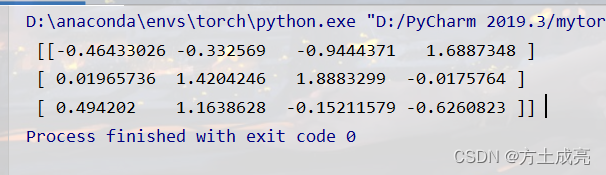sample函数是box类自带的采样函数，可以对数据进行随机的采样（采样符合正太分布，数据的上限与下限自己定义）

## 第一种用法（不对数据sample）

``````from gym import spaces
a=spaces.Box(low=-1,high=2,shape=(3,4))
print("\r",a,end=" ")
``````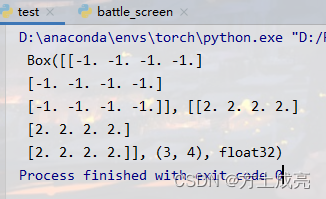## 第二种用法（对数据sample）

``````import numpy as np
from gym import spaces
a=spaces.Box(low=np.array([-1, -2]), high=np.array([2.0, 4.0]), dtype=np.float32)
for i in range(10):
print(a.sample())
``````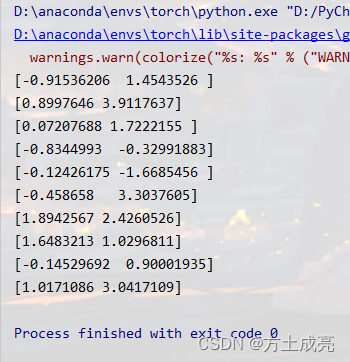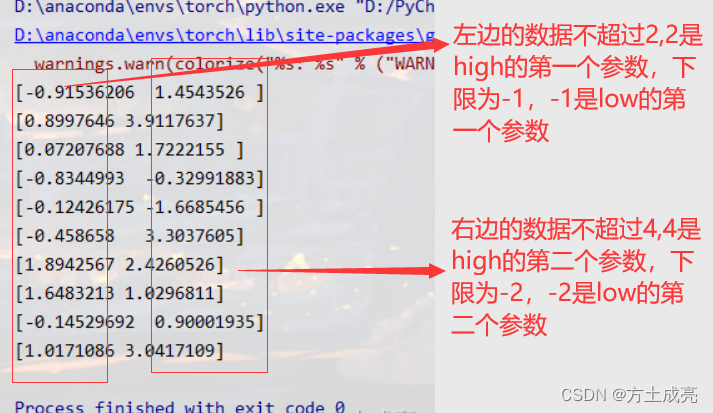## 第二种用法（不对数据sample）

``````import numpy as np
from gym import spaces
a=spaces.Box(low=np.array([-1, -2]), high=np.array([2.0, 4.0]), dtype=np.float32)
print(a)
``````